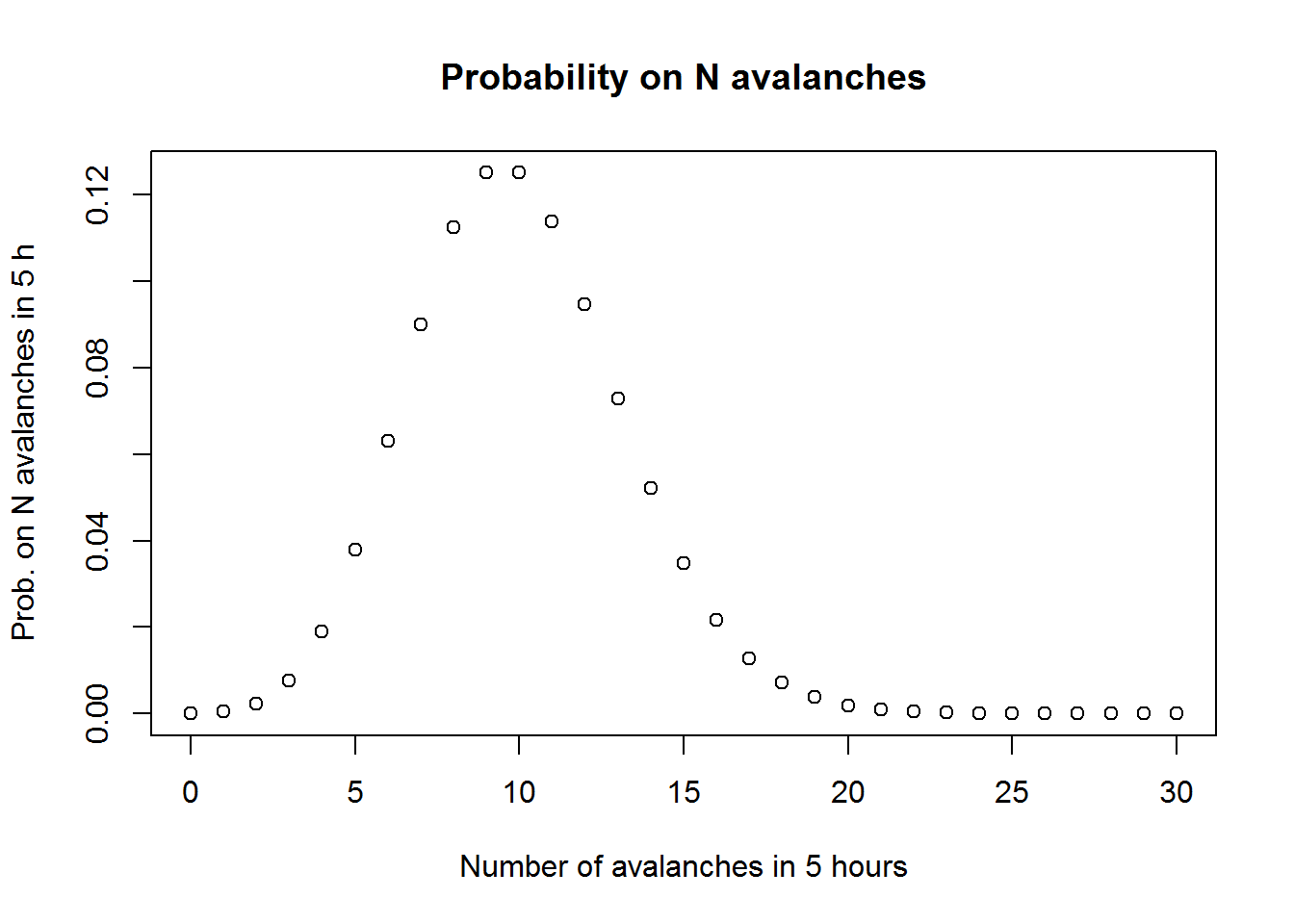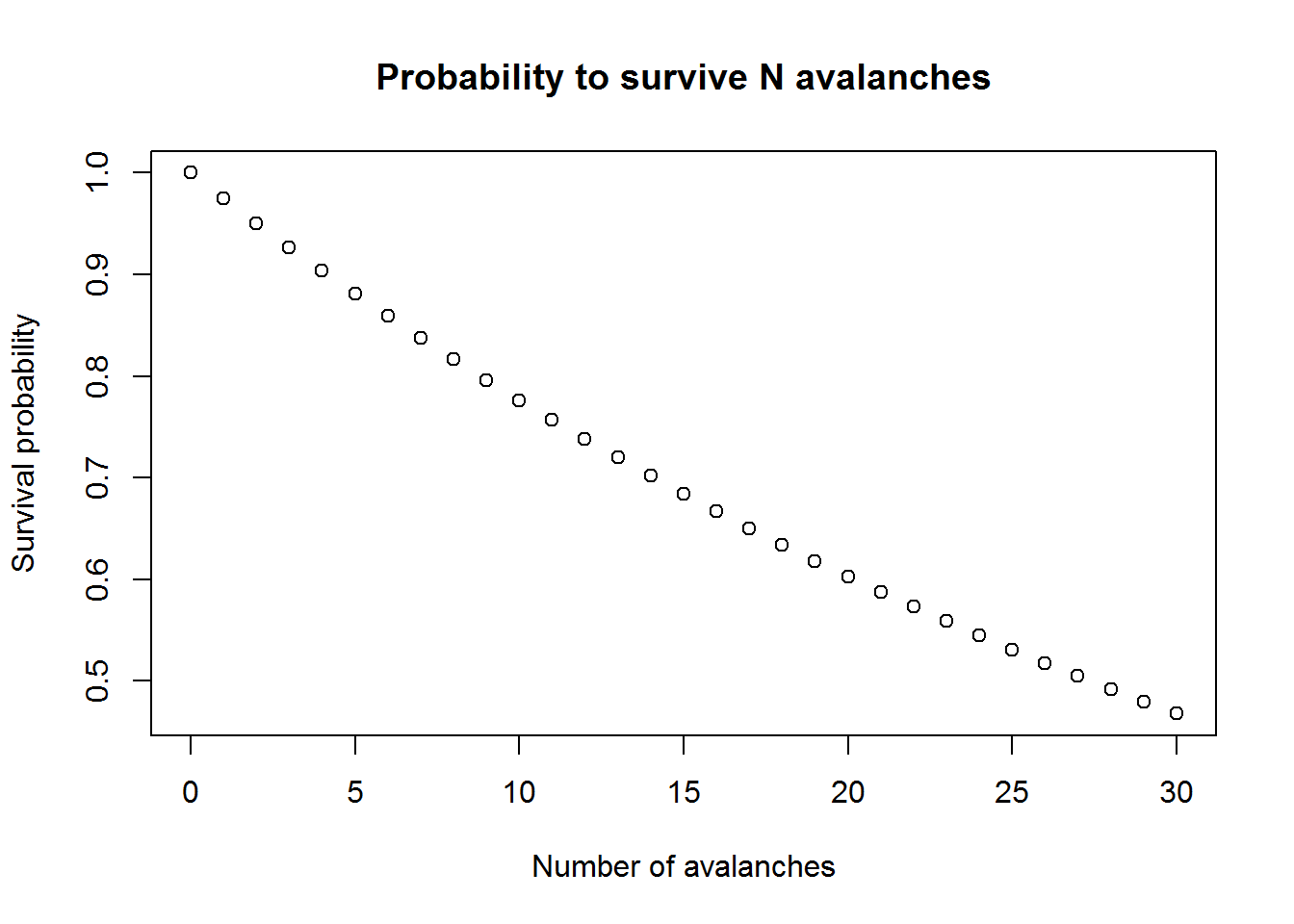## Input data

width_aval <- 0.200       # avalanche width (km)
length_valley <- 8        # valley length in (km)
lambda <- 2               # frequency: avalanches per hours
t_aval <- 30 / 3600       # time that an avalanche blocks the trail
t_trip <- 5               # trip time (h)
t_up <- t_trip * 0.8      # time to climb up (h)
t_down <- t_trip * 0.2    # time to ski down (h)

## A simple solution

First the probability not to be hit by an avalanche is calculated. You survive the whole trip when you’re not hit by neither of the 10 avalanches that occur.

# Survival probability given an avalanche occurs: avalanche width / valley length
P_surv <- 1 - width_aval/length_valley
# for the expected amount of avalanches (lambda, 10 in this case)
P_surv_E <- P_surv^(lambda*t_trip)
# of the probability to die
P_death <- 1 - P_surv_E
print(paste0("Probability to die: ", P_death))
##  "Probability to die: 0.223670379143562"

## A bit more complex solution

Avalanche occurence is a Poission process. The expected number of avalanches in 5 hours is 10. But it is possible that more or less avalanches come down in the same period. The survival probability of N avalanches weighted by the probability that N avalanches occur gives a more accurate estimate.

# Probability on N avalanches to occur in 5 hours
N.aval <- 0:30
P_N.aval <- exp(-lambda*t_trip) * (lambda*t_trip)^N.aval / factorial(N.aval)
plot(N.aval, P_N.aval,
main = "Probability on N avalanches",
xlab = "Number of avalanches in 5 hours",
ylab = "Prob. on N avalanches in 5 h")plot(N.aval, P_surv^N.aval,
main = "Probability to survive N avalanches",
xlab = "Number of avalanches",
ylab = "Survival probability")# combine the probability on N avalanches and the probability to survive them all
P_death <- 1 - sum(P_N.aval * P_surv^N.aval)
print(paste0("Probability to die: ", P_death)) # not very different of the previous result
##  "Probability to die: 0.221199252954851"

## A too complex solution

So far the time that the avalanche runs over the track was not taken into account. It can happen that an avalanche occurs in front of the Guru and he skis into it. We assume here that does not look around or tries to avoid a falling avalanche. He’s crazy anyway.

speed_up <- length_valley / t_up    # uphill speed (m/s)
speed_down <- length_valley / t_down    # uphill speed (m/s)
# effective width of an avalanche: the width is increased by the distance the Guru can cover in 30 seconds
w_eff_up <- width_aval + t_aval * speed_up
w_eff_down <- width_aval + t_aval * speed_down
# The up and downhill survival probabilities become
P_surv_up <- 1 - w_eff_up/length_valley
P_surv_down <- 1 - w_eff_down/length_valley

# simple: survive 8 avalanches during the climb and 2 on the descent
P_death <- 1 - P_surv_up^(lambda*t_up) * P_surv_down^(lambda*t_down)
print(paste0("Probability to die: ", P_death))
##  "Probability to die: 0.249831831722027"
# and taking into account the Poisson character of avalanches
# The avalanche frequencies
lambda_up <- lambda * t_up
lambda_down <- lambda * t_down
# The pdf of the number of avalanches
P_N.aval_up <- exp(-lambda_up) * lambda_up^N.aval / factorial(N.aval)
P_N.aval_down <- exp(-lambda_down) * lambda_down^N.aval / factorial(N.aval)
# Prob to survive the climb and the descent
P_surv_climb <- sum(P_N.aval_up * P_surv_up^N.aval)
print(paste0("Probability to survive the climb: ", P_surv_climb))
##  "Probability to survive the climb: 0.805198323790827"
P_surv_descent <- sum(P_N.aval_down * P_surv_down^N.aval)
print(paste0("Probability to survive the descent: ", P_surv_descent))
##  "Probability to survive the descent: 0.935506985031618"
# It's good to be fast!!"
# to survive the trip you have to survive the climb and the descent
P_surv_trip <- P_surv_climb * P_surv_descent
# and the probability to die...
print(paste0("Probability to die: ", 1-P_surv_trip)) # not very different of the previous
##  "Probability to die: 0.246731343757931"